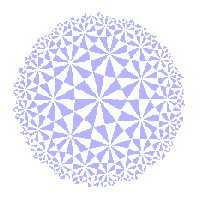# The Klein View of Geometry

The Fundamental Theorem of Inversive Geometry

 In euclidean, similarity and projective geometry, two points are sufficient to determine a line. In inversive geometry, there are infinitely many i-lines through two given points. If A, B ε E, there is one extended line through A and B, but an infinite family of circles. Here, we show that it needs three points of E+ to determine an i-line. We shall also show that, if L and M are i-lines, then there is a t in I(2) which maps L to M. Thus, the i-lines form a single i-congruence class. The key is The Fundamental Theorem of Inversive Geometry If L = (α,β,γ) and L' = (α',β',γ') are lists of distinct points of E+, then there is a unique t in I+(2) which maps L to L'. Note that the element t lies in the subgroup I+(2). There are several ways to prove this result. We offer a constructive proof, based on The (0,1,∞) Theorem If L = (α,β,γ) is a list of distinct points of E+, then there is a unique t in I+(2) which maps L to (0,1,∞). The (0,1,∞) Theorem allows us to prove the promised results about i-lines. We begin with a special case: The points 0, 1 and ∞ lie on R, the extended real axis. Since any i-line through ∞ must be an extended line, R is the only i-line through these points. Theorem I3 (1) If α, β, γ are distinct points of E+, then there is a unique i-line through these points. (2) If L and M are i-lines, then there is an inversive transformation t mapping L to M. Proof (1) By the (0,1,∞) Theorem, there is an s ε I+(2) mapping (α,β,γ) to (0,1,∞). If L is any i-line through α,β,γ, then s(L) is an i-line through 0,1,∞. By the remark before the theorem, we must have s(L) = R. Thus, s-1(R) is the unique i-line through α, β, γ. (2) If we choose a list L of three distinct points of L, and a list M of three distinct points of M. Then, by the Fundamental theorem, there is an inversive transformation t which maps L to M. Then t maps L to an i-line through the points of M. By part (1), this must be M. If we ignore the point ∞ we get an unusual proof of a standard euclidean result: Euclidean Theorem If A, B and C are distinct points of E, then either A, B and C are collinear, or they lie on a unique circle. Proof By Theorem I3, there is a unique i-line L through A, B and C. If L is an extended line, then the points are collinear. Otherwise it is a circle.